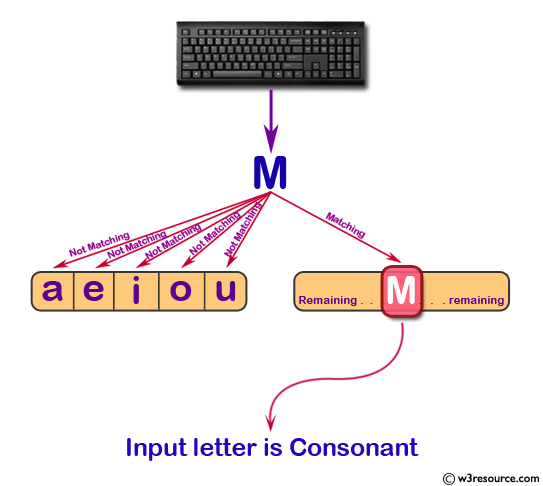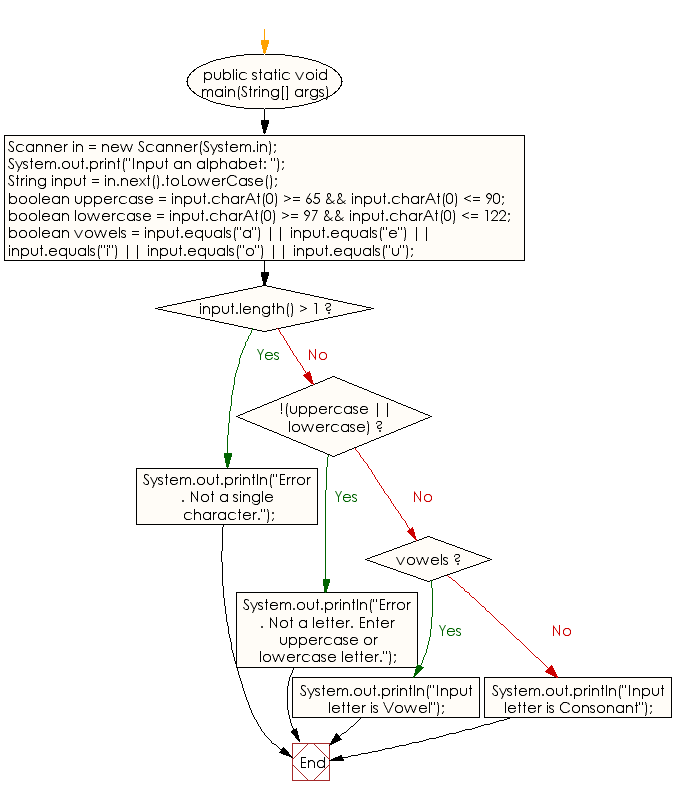﻿ Java - Find Vowel or Consonant

# Java: Find Vowel or Consonant

## Java Conditional Statement: Exercise-8 with Solution

Write a Java program that requires the user to enter a single character from the alphabet. Print Vowel or Consonant, depending on user input. If the user input is not a letter (between a and z or A and Z), or is a string of length > 1, print an error message.

Test Data
Input an alphabet: p

Pictorial Presentation:Sample Solution:

Java Code:

``````import java.util.Scanner;
public class Exercise8 {

public static void main(String[] args)
{
Scanner in = new Scanner(System.in);

System.out.print("Input an alphabet: ");
String input = in.next().toLowerCase();

boolean uppercase = input.charAt(0) >= 65 && input.charAt(0) <= 90;
boolean lowercase = input.charAt(0) >= 97 && input.charAt(0) <= 122;
boolean vowels = input.equals("a") || input.equals("e") || input.equals("i")
|| input.equals("o") || input.equals("u");

if (input.length() > 1)
{
System.out.println("Error. Not a single character.");
}
else if (!(uppercase || lowercase))
{
System.out.println("Error. Not a letter. Enter uppercase or lowercase letter.");
}
else if (vowels)
{
System.out.println("Input letter is Vowel");
}
else
{
System.out.println("Input letter is Consonant");
}
}
}
```
```

Sample Output:

```Input an alphabet: P
Input letter is Consonant
```

Flowchart:Java Code Editor:

What is the difficulty level of this exercise?

Test your Programming skills with w3resource's quiz.

﻿

## Java: Tips of the Day

IsPowerOfTwo

Checks if a value is positive power of two.

To understand how it works let's assume we made a call IsPowerOfTwo(4).

As value is greater than 0, so right side of the && operator will be evaluated.

The result of (~value + 1) is equal to value itself. ~100 + 001 => 011 + 001 => 100. This is equal to value.

The result of (value & value) is value. 100 & 100 => 100.

This will value the expression to true as value is equal to value.

```public static boolean isPowerOfTwo(final int value) {
return value > 0 && ((value & (~value + 1)) == value);
}
```

Ref: https://bit.ly/3sA5d4I

We are closing our Disqus commenting system for some maintenanace issues. You may write to us at reach[at]yahoo[dot]com or visit us at Facebook